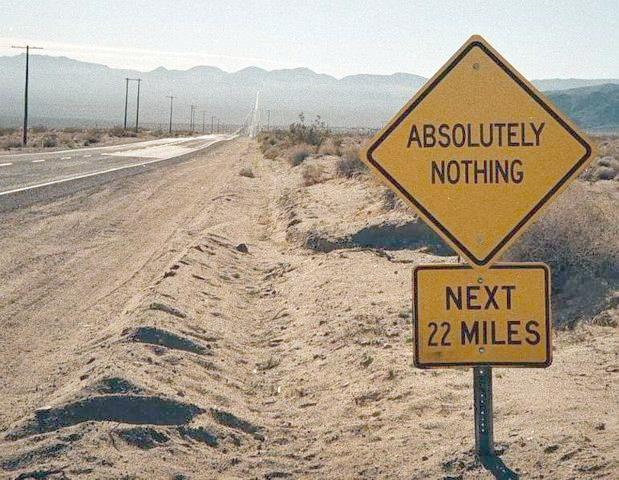# Do you know something of nothing?

Probability Level 2Calculate |$\mathcal{P}(\mathcal{P}(A))$| if A is the Empty Set and $\mathcal{P}(X)$ means "the power set of set $X$."

×

Problem Loading...

Note Loading...

Set Loading...